Business

# The Best Online Calculators to Solve Any ProblemA calculator is for more than just solving basic math problems. You can rely on online calculators to figure out scientific programs, determine loan amounts, and much more. The key is finding the right calculator for the right problem.

A proper calculator can cost hundreds of dollars, but many of these online calculators are completely free to use. This list compiles the best online calculators for a variety of different applications to help you make the right choice.## The Best Online Scientific Calculators

These calculators can be used for everything from advanced mathematics to scientific equations.

#### Desmos Scientific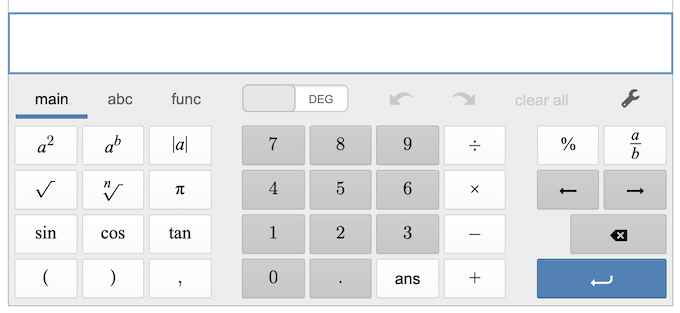Desmos is a free-to-use calculator that provides easy access to primary functions, variables, and trigonometric calculations. You can control it through both the keyboard and with a mouse.

#### Meta CalculatorThe Meta Calculator contains all of the basic functions, but includes a few features that make it stand out from the crowd. One of these is the ability to calculate the least common multiple, as well as an equation solver that lets you input up to six equations. You can also store calculations for future use.

#### GeoGebra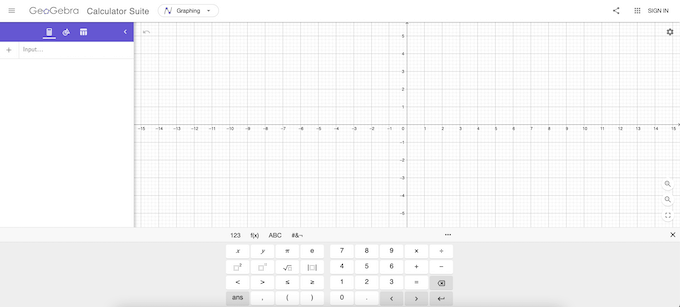GeoGebra offers a variety of math-focused calculators to solve graphing problems, geometry, and much more. It displays a graph on screen to help you see exactly how the equation plays out. You can also choose between function input, variables, and much more.

## Best Online Calculators For Loans

If you plan to get a loan for a house or car, use one of these online calculators to help determine what your overall interest will be.

#### BankrateBankrate is one of the foremost companies in the field. Just enter your loan amount, term, and interest rate per year to get an estimate of how much you will pay in interest.

#### NerdwalletNerdwallet is a major destination for people seeking financial information, so it’s not surprising it would have one of best loan calculators. Just enter the amount, the term in either years or months, interest rate, and type of loan to get an estimate of the amount you will pay.

#### CreditKarmaCreditKarma is good for more than just catchy TV jingles. The website has a loan calculator that takes the normal variables into account, but also your credit score. This gives you a more accurate estimate for what you’ll pay in interest and principal over the years.

## The Best Online Calculators for Retirement

If you plan to retire, you need to know what kind of monthly cash flow to expect.

#### Fidelity Retirement CalculatorFidelity offers one of the most effective retirement calculators. It asks you six basic questions to help determine the current state of your retirement policy and tells you how much you need per month to maintain your current way of life, as well as what you should invest each month.

#### Ultimate Retirement CalculatorThe Ultimate Retirement Calculator requests a huge amount of information, such as your age, when you plan to retire, and even your life expectancy. It then asks about your current savings, what your desired annual retirement income is, and projects the amount you will need to invest each month to reach that standard of living.

## Best Online Calculators for Statistics

If you need to calculate the statistics of a sample or set of data, try one of these online calculators.

#### CalculatorSoup Statistics Calculator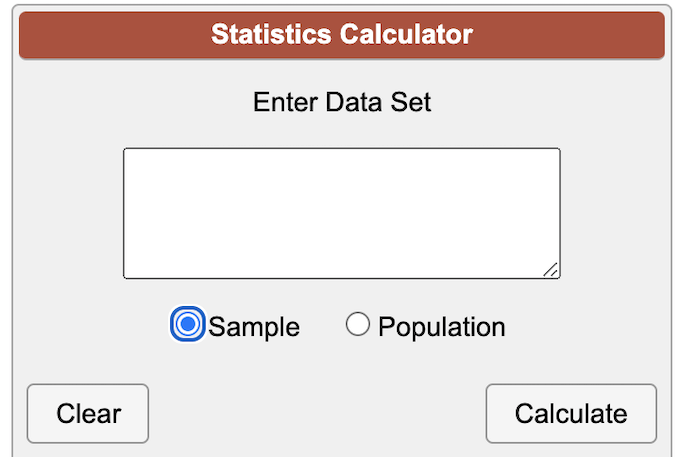This calculator is simple and straightforward. Choose whether the data set is a sample or a population and enter the information. It also provides equations regarding how to find the minimum, the maximum, and more.

#### Good Calculators Statistics Calculator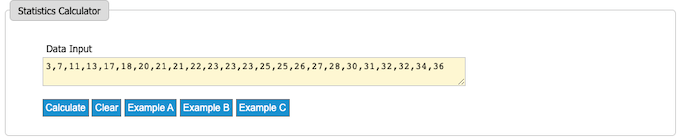The Good Calculators Statistics Calculator is a much more in-depth and complex calculator, but it provides a pie chart that demonstrates the top values once the data has been processed. If you have a complicated statistics problem, this is the best option.

## Best Online Calculator for Calories

Trying to lose weight or bulk up? Try one of these calculators to determine how many calories you need (or don’t need!)

#### FreeDieting Caloric CalculatorThis calculator makes it easy to determine how many calories you need per day to either lose weight, gain weight, or maintain. Just enter your age, gender, current weight, height, and exercise level. You can also choose which formula you want to use to perform the calculations, as well as whether you want results in calories or kilojoules.

#### MyFitnessPal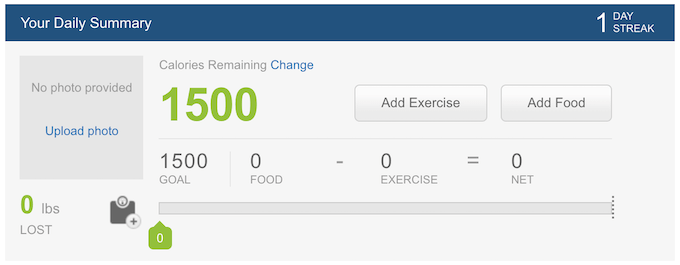MyFitnessPal is more than just a calculator–it’s an entire platform where you can track your calories, exercise, and more. The calorie tracking tool helps to automatically determine how many calories you’ve eaten and even the macronutrients you’ve taken in.

## Best Online Calculators for Algebra

Algebra and math sometimes feel like two different fields. Calculus is difficult due to the amount of algebra included. If you need some help, try one of these online calculators.

#### Symbolab Algebra Calculator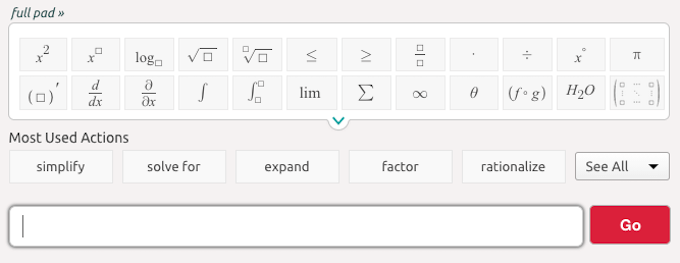The Symbolab Algebra Calculator makes it easy to perform many advanced algebraic tasks. The calculator has a quick-access tab for the most used actions like simplify, solve for, and other common word-problem terms, as well as an equation builder.

#### Math Papa Algebra Calculator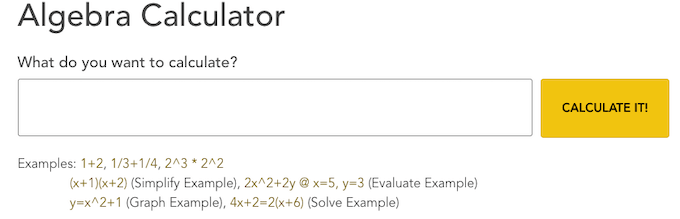The Math Papa algebra calculator is the best choice for anyone that wants to depict algebra in the most simplified terms possible. Rather than using complex equations and symbols, just type out the equation and receive a step-by-step explanation of how to solve it.

## Best Online Calculators for Trigonometry

Trigonometry can be difficult, especially if you aren’t confident in your geometry skills. These trigonometry calculators will help you solve for sine everytime.

#### Step-by-Step Trigonometry Calculator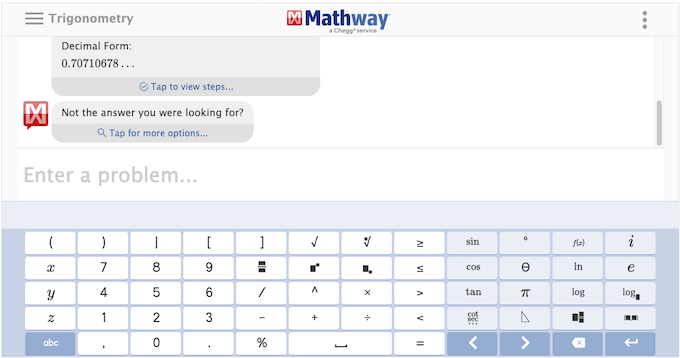One of the key components of trigonometry is the multiple steps to the equations. If you do not have the right answer at one step, you’ll mess up the rest. This calculator walks you through how to solve almost any trigonometry problem and explains how to do it. It’s like an online tutor.

#### Microsoft Math Solver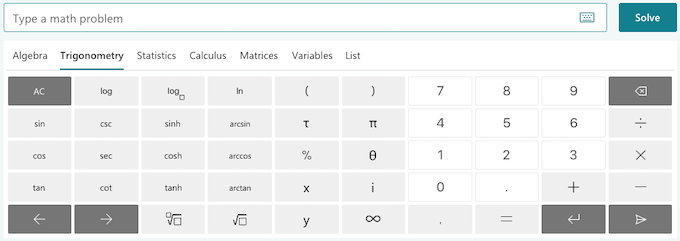This calculator not only solves trigonometry problems, but it also explains the concepts and provides videos you can watch to help you better understand the math. It’s an easy to use calculator, too–just click the operations and enter the numbers.

When faced with math, it’s a good idea to know how to do it without a calculator, but you can certainly use a computer to help you double-check your answers.